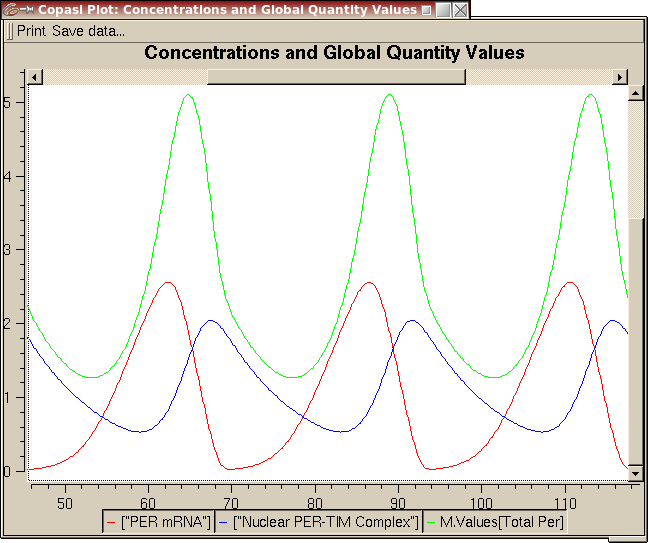## Leloup1999_CircClockModel Identifier
BIOMD0000000021
Short description
Format
SBML (L2V1)
Related Publication
• Chaos and birhythmicity in a model for circadian oscillations of the PER and TIM proteins in drosophila• Leloup JC, Goldbeter A
• Journal of theoretical biology , 6/ 1999 , Volume 198 , pages: 445-459 , PubMed ID: 10366496
• Unite de Chronobiologie Theorique, Faculte des Sciences, Universite Libre de Bruxelles, Campus Plaine, C.P. 231, B-1050 Brussels, Belgium.
• In Drosophila, circadian oscillations in the levels of two proteins, PER and TIM, result from the negative feedback exerted by a PER-TIM complex on the expression of the per and tim genes which code for these two proteins. On the basis of these experimental observations, we have recently proposed a theoretical model for circadian oscillations of the PER and TIM proteins in Drosophila. Here we show that for constant environmental conditions this model is capable of generating autonomous chaotic oscillations. For other parameter values, the model can also display birhythmicity, i.e. the coexistence between two stable regimes of limit cycle oscillations. We analyse the occurrence of chaos and birhythmicity by means of bifurcation diagrams and locate the different domains of complex oscillatory behavior in parameter space. The relative smallness of these domains raises doubts as to the possible physiological significance of chaos and birhythmicity in regard to circadian rhythm generation. Beyond the particular context of circadian rhythms we discuss the results in the light of other mechanisms underlying chaos and birhythmicity in regulated biological systems. Copyright 1999 Academic Press.
Contributors
Submitter of the first revision: Nicolas Le Novère
Submitter of this revision: Nicolas Le Novère

is
BioModels Database MODEL6617834203
BioModels Database BIOMD0000000021
KEGG Pathway dme04710
isDerivedFrom
BioModels Database BIOMD0000000171
isDescribedBy
PubMed 10366496
hasTaxon
isVersionOf

Curation status
Curated

Tags

#### Connected external resources

SBGN view in Newt Editor

Name Description Size Actions

### Model files

• Model originally submitted by : Nicolas Le Novère
• Submitted: Sep 13, 2005 2:24:15 PM
##### Revisions

(*) You might be seeing discontinuous revisions as only public revisions are displayed here. Any private revisionsof this model will only be shown to the submitter and their collaborators.

Legends
: Variable used inside SBML models

Species
Reactions
Reactions Rate Parameters
=> Mt; Cn Cell*V_sT*K_IT^n/(K_IT^n+Cn^n) K_IT=1.0; n=4.0; V_sT=1.0
P2 => P1 Cell*V_4P*P2/(K_4P+P2) K_4P=2.0; V_4P=1.0
Mt => Cell*k_d*Mt+Cell*V_mT*Mt/(K_mT+Mt) V_mT = 0.7; K_mT=0.2; k_d=0.01
P2 + T2 => CC Cell*k3*P2*T2-Cell*k4*CC k3=1.2; k4=0.6
P0 => P1 Cell*V_1P*P0/(K1_P+P0) V_1P=8.0; K1_P=2.0
T2 => Cell*k_d*T2+Cell*V_dT*T2/(K_dT+T2) V_dT = 2.0; K_dT=0.2; k_d=0.01
T1 => T2 Cell*V_3T*T1/(K_3T+T1) K_3T=2.0; V_3T=8.0
CC => Cn Cell*k1*CC-compartment_0000002*k2*Cn k1=0.6; k2=0.2
=> Mp; Cn Cell*v_sP*K_IP^n/(K_IP^n+Cn^n) v_sP=1.0; K_IP=1.0; n=4.0
Mp => Cell*k_d*Mp+Cell*V_mP*Mp/(K_mP+Mp) V_mP=0.7; K_mP=0.2; k_d=0.01
T1 => Cell*k_d*T1 k_d=0.01
T1 => T0 Cell*V_2T*T1/(K_2T+T1) V_2T=1.0; K_2T=2.0
P1 => P2 Cell*V_3P*P1/(K_3P+P1) V_3P=8.0; K_3P=2.0Curator's comment:
(added: 19 Oct 2006, 21:49:27, updated: 19 Oct 2006, 21:49:27)
Figure 2A reproduced by COPASI 4 build 19